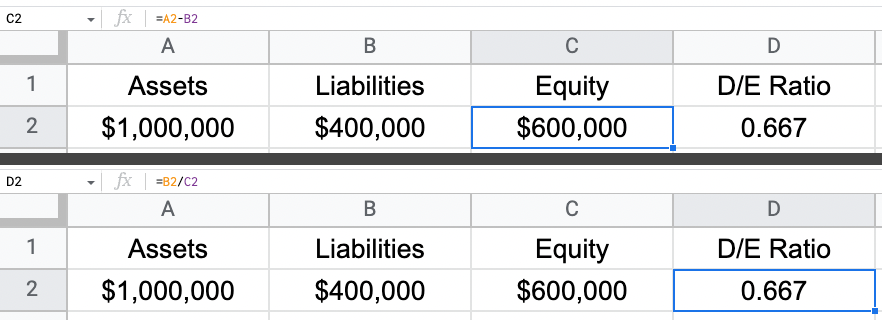# Debt-to-Equity Ratio Calculator – D/E Formula

Calculate a debt-to-equity ratio using the calculator below. Keep reading to learn more about D/E and see the debt-to-equity ratio formula.

\$
\$

## Debt to Equity (D/E) Ratio:

Learn how we calculated this below

## How to Calculate Debt-to-Equity Ratio

The debt-to-equity ratio (D/E) measures the amount of liability on a company’s balance sheet relative to the amount of shareholder’s equity on the balance sheet. D/E calculates the amount of leverage a company has, and the higher liabilities are relative to shareholder’s equity, the more leveraged the company is.

Debt can have its upside because it is cheaper than equity and doesn’t involve giving away a piece of the company. But, if debt gets too high, then the interest payments can be a severe burden on a company’s bottom line.

A company with a D/E ratio greater than 1 means that liabilities are greater than shareholder’s equity. A D/E ratio less than 1 means that shareholder’s equity is greater than total liabilities.

### Debt-to-Equity Ratio Formula

The debt-to-equity ratio is calculated using the formula below.

D/E ratio = total liabilities / shareholders’ equity

Both of these values can be found on a company’s balance sheet, which is a financial statement that details the balances for each account. The sum of liabilities and shareholder’s equity equals total assets. All assets are either financed using debt (liabilities) or equity.

Our return on equity calculator divides net income by equity instead of dividing liabilities by equity. Net income is found on a separate financial statement: the income statement. This shows the business owners how much profit the company made during a year relative to the balance of its shareholder’s equity.

For example, let’s assume we are looking at a company’s balance sheet with total assets of \$1,000,000 and liabilities of \$400,000. Using these two amounts, can we solve for the debt-to-equity ratio?

We have the amount for debt, but we first need to solve for equity. We know that liabilities plus equity equals total assets. Therefore, equity must equal \$600,000 (\$1,000,000 – \$400,000). We can now solve for the D/E ratio, which is \$400,000 / \$600,000 = 0.67.

But, what would happen if the company changes something on its balance sheet? Let’s look at two examples, one in which the company adds debt and one in which the company adds equity to the balance sheet.

If a company takes out a loan for \$100,000, then we would expect its D/E ratio to increase. Our company now has \$500,000 in liabilities and still has \$600,000 in shareholder’s equity. Assets have increased to \$1,100,000 due to the additional cash received from the loan.

In this example, the D/E ratio has increased to 0.83, which is found by dividing \$500,000 by \$600,000.

If, on the other hand, equity had instead increased by \$100,000, then the D/E ratio would fall. In this case it would fall to 0.57 (\$400,000 / \$700,000).

You can also find these results using a ratio calculator.

## How to Calculate a D/E Ratio using Excel or Google Sheets

The D/E ratio can be calculated the same way in either Microsoft Excel or Google Sheets. Enter in the amounts for total assets, liabilities, and shareholder’s equity.

After that, simply divide the liabilities by shareholder’s equity. In a cell in the spreadsheet, type: =[Liability cell] / [Shareholder’s Equity cell]. The image below shows exactly how this is done with the formula in the top right. Liabilities are in cell E1 and shareholder’s equity in cell E2.## How is the Debt-to-Equity Ratio Used?

The debt-to-equity ratio is primarily used by companies to determine its riskiness. If a company has a high D/E ratio, it will most likely want to issue equity as opposed to debt during its next round of funding. If it issues additional debt, it will further increase the level of risk in the company.

If a bank is deciding to give this company a loan, it will see this high D/E ratio and will only offer debt with a higher interest rate in order to be compensated for the risk. The interest payments will be higher on this new round of debt and may get to the point where the business isn’t making enough profit to cover its interest payments.

So, a company with high levels of debt will want to issue equity. The business owners will have to give up a portion of the business, but this allows it to bring cash into the business without increasing its interest payments.

On the other hand, a company with a very low D/E ratio should consider issuing debt if it needs additional cash. This business is seen as safer and better able to pay back its loan.

The bank will see it as having less risk and therefore will issue the loan with a lower interest rate. This company can then take advantage of its low D/E ratio and get a better rate than if it had a high D/E ratio.